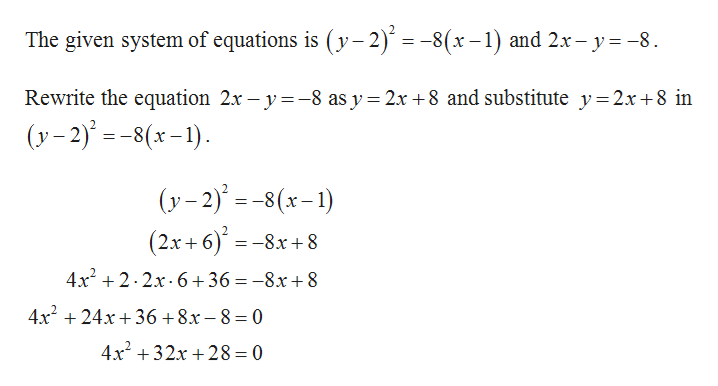# For Exercise, solve the system of nonlinear equations.(y-2)2 =-8(x-1)2x-y=-8

Question
2 views

For Exercise, solve the system of nonlinear equations.

(y-2)=-8(x-1)

2x-y=-8

check_circle

Step 1help_outlineImage TranscriptioncloseThe given system of equations is (y– 2) = -8(x– 1) and 2x- y= -8. Rewrite the equation 2x – y=-8 as y= 2x +8 and substitute y= 2x+8 in (y- 2) = -8(x–1). (y- 2) = -8(x-1) (2x+ 6)' =-8x+8 4x + 2. 2x. 6+36 = -8x+8 4x + 24x + 36 +8x – 8 = 0 4x +32x + 28=0 fullscreen

### Want to see the full answer?

See Solution

#### Want to see this answer and more?

Solutions are written by subject experts who are available 24/7. Questions are typically answered within 1 hour.*

See Solution
*Response times may vary by subject and question.
Tagged in

### Other